# Implementing Agglomerative Clustering using Sklearn

Prerequisites: Agglomerative Clustering
Agglomerative Clustering is one of the most common hierarchical clustering techniques.
Dataset – Credit Card Dataset.

Assumption: The clustering technique assumes that each data point is similar enough to the other data points that the data at the starting can be assumed to be clustered in 1 cluster.

Step 1: Importing the required libraries

 `import` `pandas as pd ` `import` `numpy as np ` `import` `matplotlib.pyplot as plt ` `from` `sklearn.decomposition ``import` `PCA ` `from` `sklearn.cluster ``import` `AgglomerativeClustering ` `from` `sklearn.preprocessing ``import` `StandardScaler, normalize ` `from` `sklearn.metrics ``import` `silhouette_score ` `import` `scipy.cluster.hierarchy as shc `

 `# Changing the working location to the location of the file ` `cd C:\Users\Dev\Desktop\Kaggle\Credit_Card ` ` `  `X ``=` `pd.read_csv(``'CC_GENERAL.csv'``) ` ` `  `# Dropping the CUST_ID column from the data ` `X ``=` `X.drop(``'CUST_ID'``, axis ``=` `1``) ` ` `  `# Handling the missing values ` `X.fillna(method ``=``'ffill'``, inplace ``=` `True``) `

Step 3: Preprocessing the data

 `# Scaling the data so that all the features become comparable ` `scaler ``=` `StandardScaler() ` `X_scaled ``=` `scaler.fit_transform(X) ` ` `  `# Normalizing the data so that the data approximately  ` `# follows a Gaussian distribution ` `X_normalized ``=` `normalize(X_scaled) ` ` `  `# Converting the numpy array into a pandas DataFrame ` `X_normalized ``=` `pd.DataFrame(X_normalized) `

Step 4: Reducing the dimensionality of the Data

 `pca ``=` `PCA(n_components ``=` `2``) ` `X_principal ``=` `pca.fit_transform(X_normalized) ` `X_principal ``=` `pd.DataFrame(X_principal) ` `X_principal.columns ``=` `[``'P1'``, ``'P2'``] `

Dendograms are used to divide a given cluster into many different clusters.

Step 5: Visualizing the working of the Dendograms

 `plt.figure(figsize ``=``(``8``, ``8``)) ` `plt.title(``'Visualising the data'``) ` `Dendrogram ``=` `shc.dendrogram((shc.linkage(X_principal, method ``=``'ward'``))) `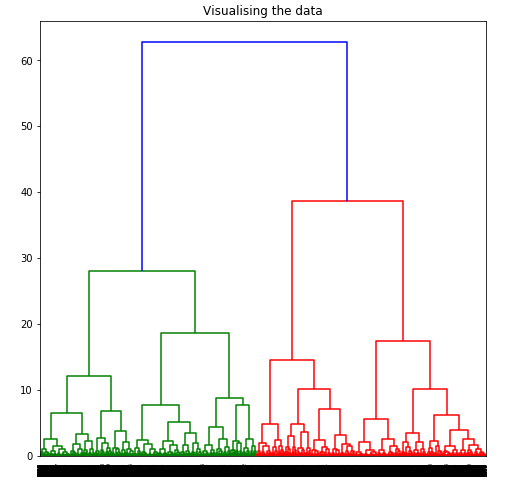To determine the optimal number of clusters by visualizing the data, imagine all the horizontal lines as being completely horizontal and then after calculating the maximum distance between any two horizontal lines, draw a horizontal line in the maximum distance calculated.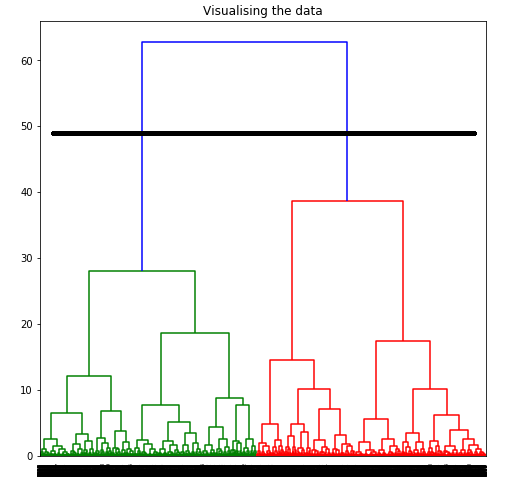The above image shows that the optimal number of clusters should be 2 for the given data.

Step 6: Building and Visualizing the different clustering models for different values of k

a) k = 2

 `ac2 ``=` `AgglomerativeClustering(n_clusters ``=` `2``) ` ` `  `# Visualizing the clustering ` `plt.figure(figsize ``=``(``6``, ``6``)) ` `plt.scatter(X_principal[``'P1'``], X_principal[``'P2'``],  ` `           ``c ``=` `ac2.fit_predict(X_principal), cmap ``=``'rainbow'``) ` `plt.show() `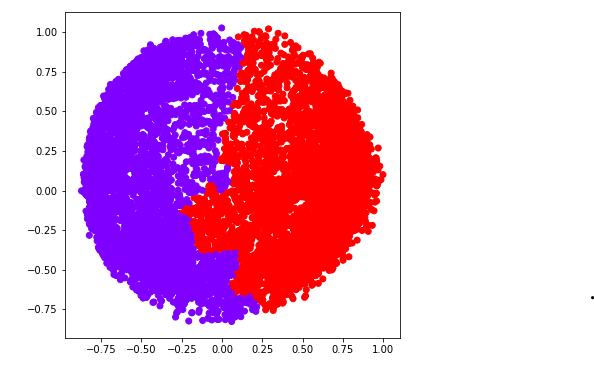b) k = 3

 `ac3 ``=` `AgglomerativeClustering(n_clusters ``=` `3``) ` ` `  `plt.figure(figsize ``=``(``6``, ``6``)) ` `plt.scatter(X_principal[``'P1'``], X_principal[``'P2'``], ` `           ``c ``=` `ac3.fit_predict(X_principal), cmap ``=``'rainbow'``) ` `plt.show() `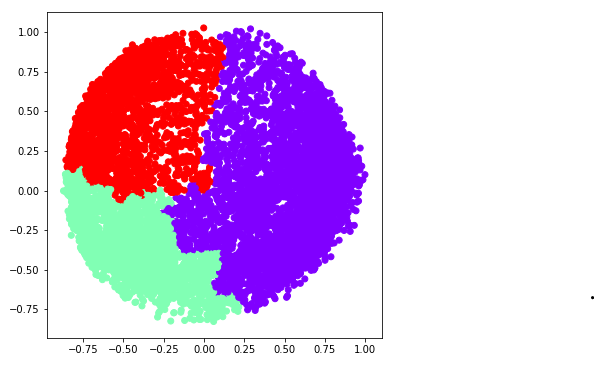c) k = 4

 `ac4 ``=` `AgglomerativeClustering(n_clusters ``=` `4``) ` ` `  `plt.figure(figsize ``=``(``6``, ``6``)) ` `plt.scatter(X_principal[``'P1'``], X_principal[``'P2'``], ` `            ``c ``=` `ac4.fit_predict(X_principal), cmap ``=``'rainbow'``) ` `plt.show() `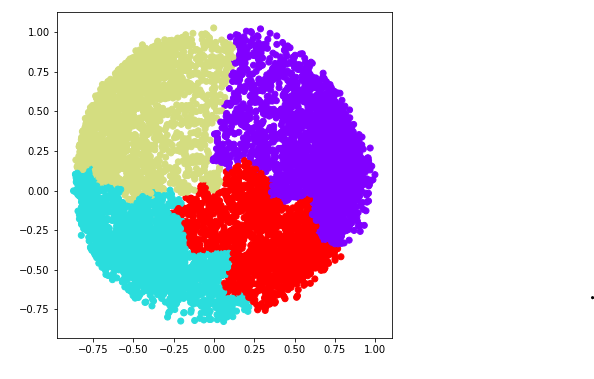d) k = 5

 `ac5 ``=` `AgglomerativeClustering(n_clusters ``=` `5``) ` ` `  `plt.figure(figsize ``=``(``6``, ``6``)) ` `plt.scatter(X_principal[``'P1'``], X_principal[``'P2'``], ` `            ``c ``=` `ac5.fit_predict(X_principal), cmap ``=``'rainbow'``) ` `plt.show() `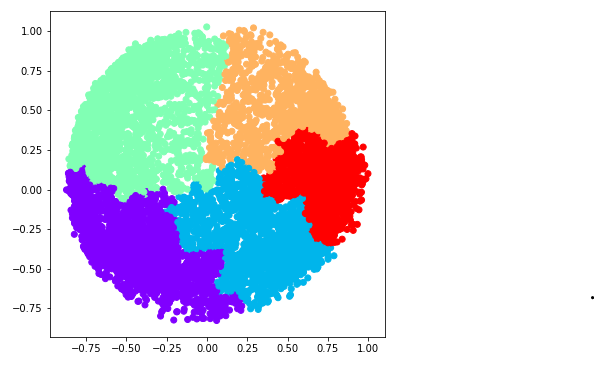e) k = 6

 `ac6 ``=` `AgglomerativeClustering(n_clusters ``=` `6``) ` ` `  `plt.figure(figsize ``=``(``6``, ``6``)) ` `plt.scatter(X_principal[``'P1'``], X_principal[``'P2'``], ` `            ``c ``=` `ac6.fit_predict(X_principal), cmap ``=``'rainbow'``) ` `plt.show() `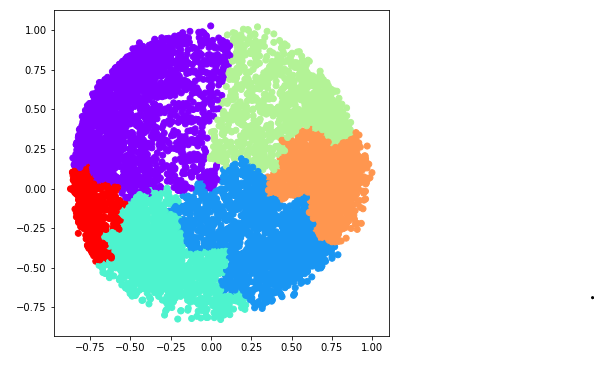We now determine the optimal number of clusters using a mathematical technique. Here, We will use the Silhouette Scores for the purpose.

Step 7: Evaluating the different models and Visualizing the results.

 `k ``=` `[``2``, ``3``, ``4``, ``5``, ``6``] ` ` `  `# Appending the silhouette scores of the different models to the list ` `silhouette_scores ``=` `[] ` `silhouette_scores.append( ` `        ``silhouette_score(X_principal, ac2.fit_predict(X_principal))) ` `silhouette_scores.append( ` `        ``silhouette_score(X_principal, ac3.fit_predict(X_principal))) ` `silhouette_scores.append( ` `        ``silhouette_score(X_principal, ac4.fit_predict(X_principal))) ` `silhouette_scores.append( ` `        ``silhouette_score(X_principal, ac5.fit_predict(X_principal))) ` `silhouette_scores.append( ` `        ``silhouette_score(X_principal, ac6.fit_predict(X_principal))) ` ` `  `# Plotting a bar graph to compare the results ` `plt.bar(k, silhouette_scores) ` `plt.xlabel(``'Number of clusters'``, fontsize ``=` `20``) ` `plt.ylabel(``'S(i)'``, fontsize ``=` `20``) ` `plt.show() `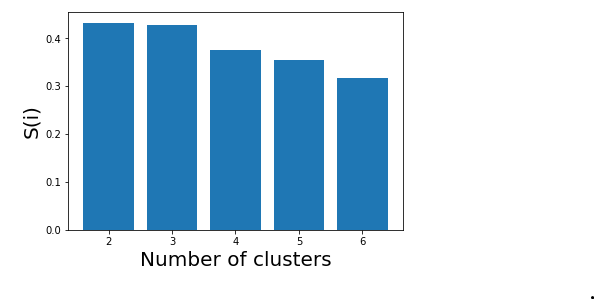Thus, with the help of the silhouette scores, it is concluded that the optimal number of clusters for the given data and clustering technique is 2.

My Personal Notes arrow_drop_upCheck out this Author's contributed articles.

If you like GeeksforGeeks and would like to contribute, you can also write an article using contribute.geeksforgeeks.org or mail your article to contribute@geeksforgeeks.org. See your article appearing on the GeeksforGeeks main page and help other Geeks.

Please Improve this article if you find anything incorrect by clicking on the "Improve Article" button below.

Article Tags :
Practice Tags :

Be the First to upvote.

Please write to us at contribute@geeksforgeeks.org to report any issue with the above content.